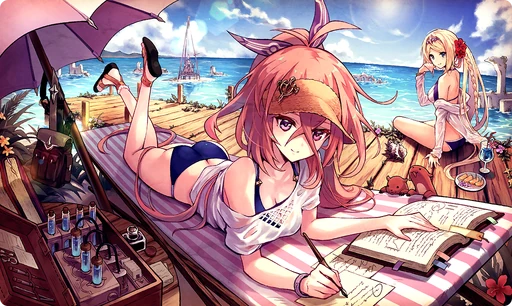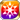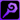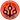# Seaside Library

Type
Equipment
Rank
5★
Max level
40
Enhancer cost
2000
Enhancer EXP
20000
Vision Clear Reward
Source
Time Limited Summon (Summer)
Release dates
• JP: 2019-08-01
• GL: 2020-06-18
AlchemistCodeDBShe is, without a doubt, incredible. However, I must say that there's really nothing splendid about investigating ruins when you're in a place like this—but I'd never actually tell her that, of course. Her voice interrupts my thoughts, "Yauras, you're not worrying yourself over needless things, are you? You really shouldn't. It isn't like you at all." Even with her sun visor, her eyes are liable to go bad...but I suppose that classifies as a needless worry, too. Come to think of it, it's even less like me to be relaxing by the sea like this, but here I am. At least I know a bit more about how to enjoy myself than she does. (Yauras' Monologue)

### Seaside Library

Raises HP by 40%/MATK by 40%/Area ATK by 10%/Max Jewels by 10%/Strong vs Greed by 30%/Strong vs Sloth by 30% for the Memento group "Holy Guard"

Target Buff (SelfSide) (CardLsSkill)
Stats
TypeMinMax
HP+40%+40%
MATK+40%+40%
Area ATK+10+10
Max Jewels+10%+10%
Strong vs <強欲>+30+30
Strong vs <怠惰>+30+30

## Stats

#### Simple stats

TypeLvl 1Lvl 30Lvl 40
MATK+10+24+30

LevelMATK
1+10
2+10
3+11
4+11
5+12
6+12
7+13
8+13
9+14
10+14
LevelMATK
11+15
12+15
13+16
14+16
15+17
16+17
17+18
18+18
19+19
20+19
LevelMATK
21+20
22+20
23+21
24+21
25+22
26+22
27+23
28+23
29+24
30+24
LevelMATK
31+25
32+25
33+26
34+26
35+27
36+27
37+28
38+28
39+29
40+30

## Group skills

### Jade Wall of the Holy Guard

#### Simple stats

TypeLvl 1Lvl 30Lvl 40
MDEF+15+33+40
Wind Res+1+3+5

#### Detailed stats

LevelMDEFWind Res
1+15+1
2+15+1
3+16+1
4+16+1
5+17+1
6+18+1
7+18+1
8+19+1
9+20+1
10+20+1
LevelMDEFWind Res
11+21+2
12+22+2
13+22+2
14+23+2
15+23+2
16+24+2
17+25+2
18+25+2
19+26+2
20+27+2
LevelMDEFWind Res
21+27+3
22+28+3
23+29+3
24+29+3
25+30+3
26+31+3
27+31+3
28+32+3
29+32+3
30+33+3
LevelMDEFWind Res
31+34+4
32+34+4
33+35+4
34+36+4
35+36+4
36+37+4
37+38+4
38+38+4
39+39+4
40+40+5

#### Limit Break

TypeLB1LB2LB3LB4LB5
MDEF+8+12+16+20+24
Wind Res+1+1+2+2+3

### Jade Wall of the Holy Guard

#### Simple stats

TypeLvl 1Lvl 30Lvl 40
MDEF+15+33+40
Wind Res+1+3+5

#### Detailed stats

LevelMDEFWind Res
1+15+1
2+15+1
3+16+1
4+16+1
5+17+1
6+18+1
7+18+1
8+19+1
9+20+1
10+20+1
LevelMDEFWind Res
11+21+2
12+22+2
13+22+2
14+23+2
15+23+2
16+24+2
17+25+2
18+25+2
19+26+2
20+27+2
LevelMDEFWind Res
21+27+3
22+28+3
23+29+3
24+29+3
25+30+3
26+31+3
27+31+3
28+32+3
29+32+3
30+33+3
LevelMDEFWind Res
31+34+4
32+34+4
33+35+4
34+36+4
35+36+4
36+37+4
37+38+4
38+38+4
39+39+4
40+40+5

#### Limit Break

TypeLB1LB2LB3LB4LB5
MDEF+8+12+16+20+24
Wind Res+1+1+2+2+3

#### Simple stats

TypeLvl 1Lvl 30Lvl 40
Magic ATK Power+3+8+10
Slash ATK Power+3+8+10
Strong vs Male+3+8+10

#### Detailed stats

LevelMagic ATK PowerSlash ATK PowerStrong vs Male
1+3+3+3
2+3+3+3
3+3+3+3
4+3+3+3
5+3+3+3
6+3+3+3
7+4+4+4
8+4+4+4
9+4+4+4
10+4+4+4
LevelMagic ATK PowerSlash ATK PowerStrong vs Male
11+4+4+4
12+4+4+4
13+5+5+5
14+5+5+5
15+5+5+5
16+5+5+5
17+5+5+5
18+6+6+6
19+6+6+6
20+6+6+6
LevelMagic ATK PowerSlash ATK PowerStrong vs Male
21+6+6+6
22+6+6+6
23+6+6+6
24+7+7+7
25+7+7+7
26+7+7+7
27+7+7+7
28+7+7+7
29+8+8+8
30+8+8+8
LevelMagic ATK PowerSlash ATK PowerStrong vs Male
31+8+8+8
32+8+8+8
33+8+8+8
34+8+8+8
35+9+9+9
36+9+9+9
37+9+9+9
38+9+9+9
39+9+9+9
40+10+10+10

#### Limit Break

TypeLB1LB2LB3LB4LB5
Magic ATK Power+2+3+4+5+6
Slash ATK Power+2+3+4+5+6
Strong vs Male+2+3+4+5+6

### Beach Observation

#### Simple stats

TypeLvl 1Lvl 30Lvl 40
HP+130+330+400
MATK+20+49+60
Strong vs Wind+5+12+15

#### Detailed stats

LevelHPMATKStrong vs Wind
1+130+20+5
2+136+21+5
3+143+22+5
4+150+23+5
5+157+24+6
6+164+25+6
7+171+26+6
8+178+27+6
9+185+28+7
10+192+29+7
LevelHPMATKStrong vs Wind
11+199+30+7
12+206+31+7
13+213+32+8
14+220+33+8
15+226+34+8
16+233+35+8
17+240+36+9
18+247+37+9
19+254+38+9
20+261+39+9
LevelHPMATKStrong vs Wind
21+268+40+10
22+275+41+10
23+282+42+10
24+289+43+10
25+296+44+11
26+303+45+11
27+310+46+11
28+316+47+11
29+323+48+12
30+330+49+12
LevelHPMATKStrong vs Wind
31+337+50+12
32+344+51+12
33+351+52+13
34+358+53+13
35+365+54+13
36+372+55+13
37+379+56+14
38+386+57+14
39+393+58+14
40+400+60+15

#### Limit Break

TypeLB1LB2LB3LB4LB5
HP+48+96+144+192+240
MATK+12+18+24+30+36
Strong vs Wind+3+4+6+7+9

#### Max Limit Break

TypeLB5
Missile ATK Power+16

## Vision abilities

### Limit Reached Bonus

#### Ability base

• Slot:Support

Revealed after Limit Reached. Raises AGI after appearing on map.

#### Ability max

• Slot:Action

Raises AGI after appearing on map.

### Scorching Mechanical Dragon - Incandescent Bindings

#### Ability base

• Slot:Action
• DMG Type:Magical
• ATK Type:Magic
• Element:Fire

Fire Mag ATK on units within area (Strong vs Wind) & raises Power for Fire units from Slothstein [Range: 4, Area: Square (3), Height Range: 2]

Skill Effect
TypeMinMax
Attack90%110%
• Charges: 1
• Jewel Cost: 75
• Height: 2
• Select Range: Diamond
• Range: 0-4
• Select Scope: Square
• Scope: 1

#### Ability max

• Slot:Action
• DMG Type:Magical
• ATK Type:Magic
• Element:Fire

Fire Mag ATK on units within area (Strong vs Wind) & raises Power for Fire units from Slothstein [Range: 4, Area: Square (3), Height Range: 2]

Skill Effect
TypeMinMax
Attack132%132%
• Charges: 1
• Jewel Cost: 75
• Height: 2
• Select Range: Diamond
• Range: 0-4
• Select Scope: Square
• Scope: 1

[[Category:Strong vs <強欲>]][[Category:Strong vs <怠惰>]]